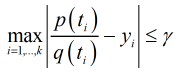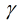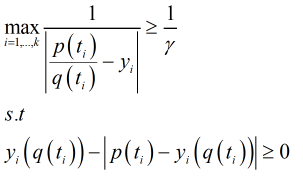# Quasiconvex problem

Hi everyone, I have an optimization problem of the form,

``````((x_1+x_2 ))/((2^(x_1/B)+2^(x_2/A)+y) ).
``````

A and B are known constants. The variables are x_1, x_2 and y. All my inequality constraint sets are convex and equality constraint sets are affine. I strongly believe this is Quasiconvex since the numerator is affine and the denominator is a convex function.

In solving it through a sequence of convex feasibility problems, I introduce a new and additional constraint of the form,

``````(x_1+x_2 )-η(2^(x_1/B)+2^(x_2/A)+y)≤0,
``````

where η is known (already solved for). Because of this new constraint I understand I cannot use CVX since

``````-η(2^(x_1/B)+2^(x_2/A)+y)
``````

is a concave function and not convex. Now my question; please is there anyway that I can express this additional constraint in a convex form??

Thank you.

Your original claim about quasiconvexity is incorrect. The ratio of an affine function and a positive concave function would be quasiconvex. But not so when the denominator is convex.

Thank you very much Sir Michael for your reply. I totally agree with you that this is nonconvex.
Ex. 6.9 is from Prof. Boyd’s Convex optimization book.

|The objective function can be rewritten as,which can be solved by firstly findingby the bisection method and then solving the feasibility programfor the variables a and b.

I solved the same problem, but with the inverse of the objective function and the constraint for the feasibility program as shown below using CVX..
And for the two cases, I had the same optimal solutions for the variables a and b.

I am not sure if what I have done above is right (solving the inverse). But if it is right, then for my optimization problem, I can take the inverse (which will be ratio of a convex function and an affine function (also positive concave) ) and write the additional constraint in a suitable form to fit the new objective function.

In the text you’ve cited from the Convex Optimization book, q(t_i) is affine, not convex/concave, and that is a critical difference.

Thank you very much. I really appreciate it.